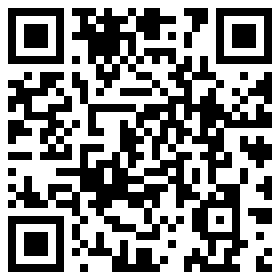| | |

|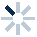|
APP端下载AndroidiPhone
|
￥24

|
2人点赞
1022人已学习
|

3天无理由退款

3天无理由退款：退款将以超级币形式退至您的超级课堂学习账户，便于您重新选购其他课程。恶意退款将被冻结账号。

• 1、​容斥原理的公式一，也被称作两量重叠问题：如果被计数的事物有A、B两类，则A或B=A+B-A且B。也可以根据韦恩图“总数，等于两类数目之和，减去重复数目”这个方式去形象记忆。
2、 有的题目在公式一的基础上还存在“非A且非B”。这种情况不过就是扩大了全体数目的范围，稍做变形可知，A且B=A+B+非A且非B-全体数目。
• 1、两种韦恩图，不重合的区域分别有三块和四块。对应的类别分别是“A且非B”、“B且非A”、“A且B”和“非A且非B”，这些区域能帮助我们进行无重复分类。
2、 我们用容斥原理解决了两道数论类的题目，它们都符合相同的韦恩图模型。
• 1、由韦恩图发现，若A且B达到最大值，为A、B中较小一类的数目，则A或B达到最小值，为A、B中较大一类的数目。非A且非B也达到最大值，为全体数目-A、B中较大一类的数目；若A且B达到最小值0，则A或B达到最大值，为A+B。非A且非B也达到最小值，为全体数目-A-B。
• 1、如果全体小于A+B，则A、B两圆无法完全分离的，总有重叠部分。此时，“A或B”的最大值就是全体数目，“非A且非B”的最小值就是0，“A且B”的最小值就是A+B-全体数目。
• 1、容斥原理公式一的面积应用：对于两个有重叠的几何图形，它们覆盖的面积等于这两个图形各自的面积再减去它们重叠部分的面积
2、 公式一的面积应用的推广：对于多个有重叠的几何图形，如果重叠部分都是由某两个图形产生，那么这些图形覆盖的面积就等于所有图形的面积和减去所有重叠部分的面积。要注意每个重叠部分只涉及两个图形时才适用于公式一的面积应用的推广
3、 掌握了这两个原理后，求有重叠的几何图形的覆盖面积就变得很容易了
• 1、三量重叠问题的公式，即容斥原理的公式二：如果被计数的事物有$A$、$B$、$C$三类，那么$A$类或$B$类或$C$类事物个数$=A$类事物个数$+B$类事物个数$-$既是$A$类又是$B$类的事物个数$-$既是$A$类又是$C$类的事物个数$-$既是$B$类又是$C$类的事物个数$-$既是$A$类又是$B$类又是$C$类的事物个数
2、 在具体计算时为了方便可以将条件分为这三类：满足某一类的、满足两类的，以及满足三类的个数。用满足某一类的个数和减去满足两类的个数和，再加上满足三类的个数，得到的就是至少满足一类的个数
3、 在三量重叠问题中，全体个数$=A$或$B$或$C$的个数加上非$A$非$B$非$C$的个数
• 1、三量重叠问题中的两层重叠分别是$A$与$B$、$A$与$C$和$B$与$C$代表的两类重叠；以及$A$、$B$、$C$的三类重叠
2、 注意这两种说法："满足$A$和$B$的"和"只满足$A$和$B$的"，满足$A$和$B$的是所有$A$、$B$重叠的部分，包含$A$、$B$、$C$重叠的部分；而只满足$A$和$B$的要从$A$、$B$重叠的部分中减掉$A$、$B$、$C$重叠的部分
3、 为了准确利用公式二可采用两种针对"只满足$A$和$B$的"这种条件的方法：补漏法与方程法，其中方程法可以配合韦恩图来操作
• 1、介绍处理三类重叠问题的四种思路，第一种是极限法：(1)当已知三种两类重叠的个数时，三类重叠的个数最大值就是其中两类重叠的最小值，而如果没有全体个数的限定，三类重叠的个数最小值就是$0$；(2)如果条件有全体个数的限定，三类重叠的个数最小值还是$0$，而最大值则需要通过公式二来计算得到，即全体个数$-$一类个数和$+$两类重叠个数和
2、 第二种是分步法：分为两步，先讨论其中两类重叠的个数，再讨论它与第三类重叠的个数
3、 第三种是逆向思考法，用于各类个数较多，不容易直接计算的问题。思路是先求出各类事物的反面个数，再求出至少满足一类的反面个数的最大值，再反过来，得到同时满足多类的个数的最小值
4、 第四种是排队法，将相应的计数问题看作包含全体个数的人来排队，并且将问题分为两类之间的重叠问题。若考虑两类重叠个数的最小值，则让这两类特征的人从两端排队，寻找中间的重叠人数；若考虑两类重叠个数的最大值，则让这两类特征的人从某一端排队，寻找这一端的重叠人数
• 综合练习

• 1晨晨cic
• 2开心锤锤
• 3超级学员2816550
• 4j_s93
• 5奶奶的手机
• 6超级学员4108890
• 7八年级2班张楠杭
• 8超级学员4228020
• 9超级学员4278545
• 10超级学员4325588227503人在学
￥ 16 ￥ 16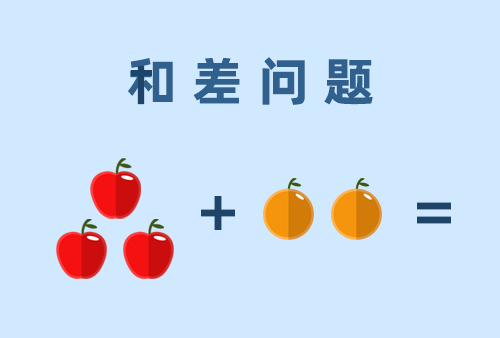224899人在学
￥ 28 ￥ 28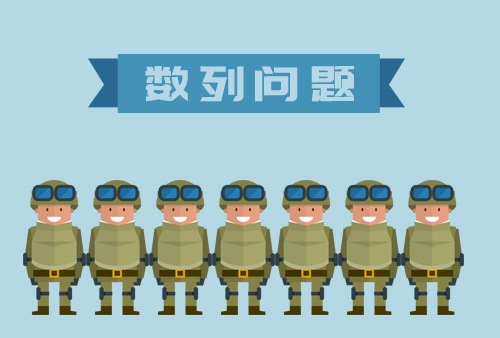219517人在学
￥ 32 ￥ 32289128人在学
￥ 0 ￥ 0

• 0
• 点击分享有好礼
•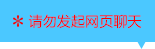•app端下载关注微信号